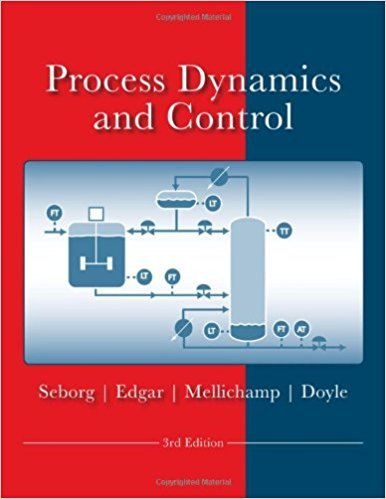×
Get Full Access to Process Dynamics And Control - 3 Edition - Chapter 5 - Problem 5.23
Get Full Access to Process Dynamics And Control - 3 Edition - Chapter 5 - Problem 5.23

×

# For a stirred-tank heater, assume the transfer functionISBN: 9780470128671 148

## Solution for problem 5.23 Chapter 5

Process Dynamics and Control | 3rd Edition

• Textbook Solutions
• 2901 Step-by-step solutions solved by professors and subject experts
• Get 24/7 help from StudySoup virtual teaching assistantsProcess Dynamics and Control | 3rd Edition

4 5 1 329 Reviews
14
1
Problem 5.23

For a stirred-tank heater, assume the transfer function between the heater input change u(t) (caUsec) and the tank temperature change y(t) COC) can be modeled as K G(s) = --1 TS + (a) Using the Final Value Theorem, find the steady-state response for a unit rectangular pulse change in the heating ( 1 -s) rate U(s) = ~ . (b) Repeat the calculation in (a) for a unit ramp ( U(s) = s 1 2). (c) For both cases (a) and (b), explain your answer physically. Is there a physical limitation on the ramping of the heating rate?

Step-by-Step Solution:

Problem 5.23For a stirred-tank heater, assume the transfer function between the heater input change u(t)(cal/sec) and the tank temperature change y(t) (°C) can be modeled as G(s) = (K/s + 1)(a) Using the Final Value Theorem, find the steady-state response for a unit rectangular pulse 1 eschange in the heating rate U(s) = s 1(b) Repeat the calculation in (a) for a unit ramp U(s) = s2 .(c) For both cases (a) and (b), explain your answer physically. Is there a physical limitation onthe ramping of the heating rate Step-by-step solution Step 1 of 3 ^(a)Consider the expression for the transfer function of the system, G(s). G(s) = K ……….(1) s + 1Write the expression for the input U(s). s U(s) = 1 e …………(2) sConsider the expression for Y(s). Y(s) = G(s) U(s) ……….(3)Substitute equations (1) and (2) in (3). K 1e s Y(s) = ………..(4) s + 1 sConsider the expression for final value theorem. ………..(5)Substitute equation (4) in (5)\nTherefore, the steady-state response of the system at input .

Step 2 of 3

Step 3 of 3

##### ISBN: 9780470128671

The answer to “For a stirred-tank heater, assume the transfer function between the heater input change u(t) (caUsec) and the tank temperature change y(t) COC) can be modeled as K G(s) = --1 TS + (a) Using the Final Value Theorem, find the steady-state response for a unit rectangular pulse change in the heating ( 1 -s) rate U(s) = ~ . (b) Repeat the calculation in (a) for a unit ramp ( U(s) = s 1 2). (c) For both cases (a) and (b), explain your answer physically. Is there a physical limitation on the ramping of the heating rate?” is broken down into a number of easy to follow steps, and 98 words. Process Dynamics and Control was written by and is associated to the ISBN: 9780470128671. Since the solution to 5.23 from 5 chapter was answered, more than 382 students have viewed the full step-by-step answer. This textbook survival guide was created for the textbook: Process Dynamics and Control, edition: 3. This full solution covers the following key subjects: change, rate, tank, unit, heating. This expansive textbook survival guide covers 24 chapters, and 420 solutions. The full step-by-step solution to problem: 5.23 from chapter: 5 was answered by , our top Engineering and Tech solution expert on 11/15/17, 04:03PM.

Unlock Textbook Solution